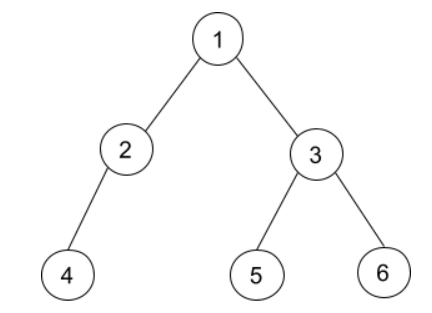# Populating Next Right Pointers in Each Node II

Posted: 23 Mar, 2021
Difficulty: Moderate

## PROBLEM STATEMENT

#### Note:

``````1. Node ‘U’ is said to be the next node of ‘V’ if and only if  ‘U’ is just next to ‘V’ in tree representation.
2. If there is no next right node, then the next pointer has to be ‘NULL’. Particularly root node and rightmost nodes have ‘next’ node equal to ‘NULL’.
3. Each node of the binary tree has three-pointers, ‘left’, ‘right’, and ‘next’. Here ‘left’ and ‘right’ are the children of a node, and ‘next’ is one extra pointer that we need to update.
``````
##### Input Format :
``````The first line contains an integer 'T' which denotes the number of test cases to be run.

The first line of each test case contains elements of the tree in the level order form. The line consists of values of nodes separated by a single space. In case a node is null, we take -1 in its place.

For example, the input for the tree is depicted in the below image.
````````````For example, the input for the tree depicted in the above image would be :

1
2 3
4 -1 5 6
-1 -1 -1 -1 -1 -1
``````

#### Explanation :

``````Level 1 :
The root node of the tree is 1

Level 2 :
Left child of 1 = 2
Right child of 1 = 3

Level 3 :
Left child of 2 = 4
Right child of 2 = null (-1)
Left child of 3 = 5
Right child of 3 =  6

Level 4 :
Left child of 4 = null (-1)
Right child of 4 = null (-1)
Left child of 5 = null (-1)
Right child of 5 = null (-1)
Left child of 6 = null (-1)
Right child of 6 = null (-1)
``````

#### Note :

``````The above format was just to provide clarity on how the input is formed for a given tree.
The sequence will be put together in a single line separated by a single space. Hence, for the above-depicted tree, the input will be given as:
1 2 3 4 -1 5 6 -1 -1 -1 -1 -1 -1
``````

#### Output Format :

``````For each test case, print the tree’s level order traversal after updating the ‘next’ for each node where  ‘-1’   denoting the null node.

The output of each test case will be printed in a separate line.
``````
##### Note :
``````You do not need to print anything. It has already been taken care of. Just implement the given function.
``````

#### Constraints :

``````1 <= T <= 5
0 <= N <= 3000
1 <= data <= 3000

Where ‘data’ is the value of the node of the binary tree.

Time Limit : 1 sec
``````Approach 1

The idea here is to use BFS, which is nothing but the level order traversal. We can maintain all nodes of the same level together by using the queue data structure. For a particular node, we start popping nodes sequentially and always update the next pointer of the just popped node with the current node.

#### Steps:

• If the root is initially NULL, then just return root.
• Create a queue to store all nodes which are going to be explored, and push root.
• Run a while loop until the queue becomes empty:
• Create a variable named previous to store the previously popped node.
• Create a variable named n to store the current queue size.
• Run a for loop from i = 0 to i = n, i.e., to traverse all nodes of the current level, in each iteration:
• Store the front node in the variable named current, and pop the node from the queue.
• If this is not the left-most node, then update the previous.next = current.
• Push the child of this node into the queue.
• Update previous with the current.
• Finally, return the root.